General Form of an Estimable Function

This section demonstrates a shorthand technique for displaying the generating set for any estimable. Suppose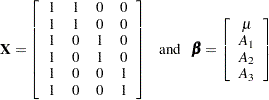is a generating set for, but so is the smaller set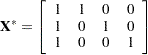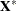is formed fromby deleting duplicate rows.

Since all estimables must be linear functions of the rows offor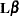to be estimable, anfor a single-degree-of-freedom estimate can be represented symbolically as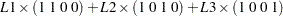or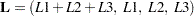For this example,is estimable if and only if the first element ofis equal to the sum of the other elements ofor if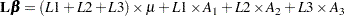is estimable for any values of
,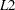, and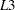.

If other generating sets forare represented symbolically, the symbolic notation looks different. However, the inherent nature of the rules is the same. For example, if row operations are performed onto produce an identity matrix in the first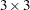submatrix of the resulting matrix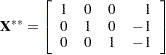then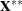is also a generating set for. An estimablegenerated fromcan be represented symbolically as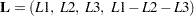Note that, again, the first element ofis equal to the sum of the other elements.

With multiple generating sets available, the question arises as to which one is the best to representsymbolically. Clearly, a generating set containing a minimum of rows (of full row rank) and a maximum of zero elements is desirable.

The generalized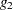-inverse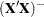of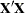computed by the modified sweep operation (Goodnight; 1979) has the property that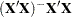usually contains numerous zeros. For this reason, in PROC GLM the nonzero rows ofare used to representsymbolically.

If the generating set represented symbolically is of full row rank, the number of symbols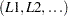represents the maximum rank of any testable hypothesis (in other words, the maximum number of linearly independent rows for anymatrix that can be constructed). By letting each symbol in turn take on the value of 1 while the others are set to 0, the original generating set can be reconstructed.